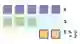# C# Modulo Examples

Apply the modulo division operator to get remainders from dividing numbers.

Modulo. This operator gets a remainder. It provides a way to execute code once every several iterations of a loop. To use modulo, we specify the percentage sign character.Modulo notes. Modulo has some unique properties. As with all low-level operations, it has a specific cost. We analyze modulo division in the C# language.DivideOdd, EvenEvery Nth Element

An example. Modulo division is expressed with the percentage sign. It is implemented with "rem" in the intermediate language. Rem takes the top 2 values on the evaluation stack.
Then: Rem performs the computation that returns the remainder of the division. It pushes that value onto the evaluation stack.
Here: This example demonstrates the math behind modulo. The expressions here are turned into constants during the C# compilation step.
5 modulo 3: When 5 is divided by 3, we have 2 left over—only one 3 can be part of 5. The modulo result is 2.C# program that uses modulo operator using System; class Program { static void Main() { // When 5 is divided by 3, the remainder is 2. Console.WriteLine(5 % 3); // When 1000 is divided by 90, the remainder is 10. Console.WriteLine(1000 % 90); // When 100 is divided by 90, the remainder is also 10. Console.WriteLine(100 % 90); // When 81 is divided by 80, the remainder is 1. Console.WriteLine(81 % 80); // When 1 is divided by 1, the remainder is zero. Console.WriteLine(1 % 1); } } Output 2 10 10 1 0

Loop example. We can use modulo in a loop for an interval or step effect. If we use a modulo operation on the loop index variable, we can execute code at an interval.
Note: This example shows how to write to the screen every 10 iterations in the for-loop.
For
Important: The 3 numbers in the condition in the if-statement can have any values, but we cannot divide by 0.
C# program that uses modulo division in loop using System; class Program { static void Main() { // // Prints every tenth number from 0 to 200. // Includes the first iteration. // for (int i = 0; i < 200; i++) { if ((i % 10) == 0) { Console.WriteLine(i); } } } } Output 0 10 20 30 40 50 60 70 80 90 100 110 120 130 140 150 160 170 180 190

Error, divide by zero. If you use modulo by 0, you will get a compile error or a runtime exception. The denominator must never be zero.DivideByZeroException
C# program that causes divide by zero error class Program { static void Main() { int zero = int.Parse("0"); int result = 100 % zero; } } Output Unhandled exception. System.DivideByZeroException: Attempted to divide by zero. at Program.Main()...

Performance. Modulo is slower than other arithmetic operators such as increment and decrement or even multiply. This is a hardware limitation on computers.
But: The total time required for individual modulo operations is tiny compared to other tasks such as disk reads or network accesses.
So: If you can reduce those operations with modulo division, you can improve overall performance.